﻿ Ab-initio Hylleraas functions of helium, lithium are applicable ?

# Nobel prize should NOT "hamper" science progress.

## If "fantasy" inflation gets Nobel prize, Science will "die".

(Fig.1) Superluminal (= fantasy ! ) inflation, multiverse get Nobel prize ? → Science will "stop".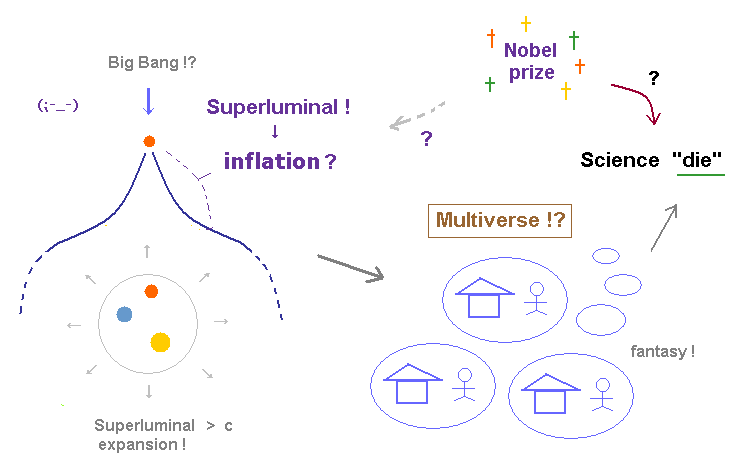Recently, we heard the news that the smoking gun evidence for cosmic inflation was found.
Though it still lacks definite proof, the present physical society tends to favor this "fantasy" inflation.

This inflation argues that our universe expanded much faster than light after Big Bang, which leads to producing "multi-universe" (= many-worlds !? ). See this site.

Unfortunately, if this inflation gets Nobel prize someday, the development of our science will end or be delayed.
If Nobel prize endorses this unrealistic inflation, physicists will come to just pursue "illusory" concepts throughout their lives.

This situation is very "dangerous" for development of our science.
It means all applied fields such as medicine and industry will be obstructed for a long time.

## The proof of inflation = B-mode ? is impossible, and due to other effects.

(Fig.2) "Smoking gun" evidence of inflation is very doubtful. It cannot survive for 13.7 billion years !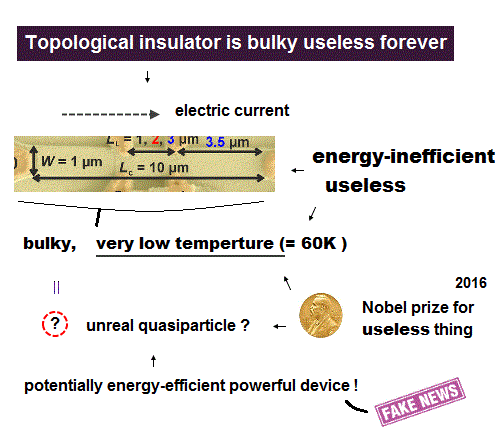As shown on this site and this page, B-mode polarized light is very faint (= only 0.4 microK ! ).
From a commonsense point of view, it is impossible that this very faint microwave is an imprint of inflation 13.7 billion years ago.

The current cosmology has to rely on strained idea that uniform microwave background (= CMB, 3 K ) and weak B-mode polarization (= 0.4 μK ) are the results of the expanding universe after Big Bang, due to denying ether-like matter.

So, this extremely faint and changeable B-mode pattern must remain as it is for 13.7 billion years (← impossible ! ) to explain CMB and inflation.   As you feel, this theory is basically unreasonable.

## Nobel committee should NOT "ignore" fatal paradoxes of relativity.

(Fig.3) Ignoring fatal paradoxes → "fantasy" inflation, SUSY were produced. → Science "die".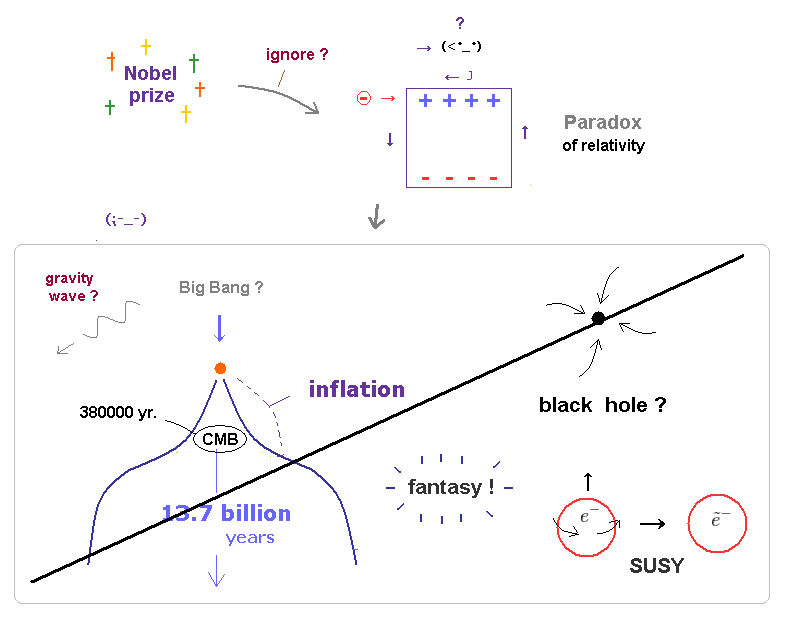Unfortunately, almost all textbooks, universities and media are concealing these important facts from new students and ordinary people.

This situation is very dangerous for newcomers in physics.
If they devote their whole life to the wrong fields based on relativity, they would surely blast their career.

All of the particle physics ( ex. Higgs ), supersymmetry, inflation, gravity-wave are based on relativistic theory.
So if special relativity includes fatal paradoxes, these theories are all wrong.

If influential Nobel prizes are given to these relativity-related theories, it's very harmful both to promising students and the development of science.

## Higgs becomes ONLY a "news item" for the media and bloggers.

(Fig.4) Heavy tax → "expensive" accelerator → Higgs gives NO benefit ( except to media ).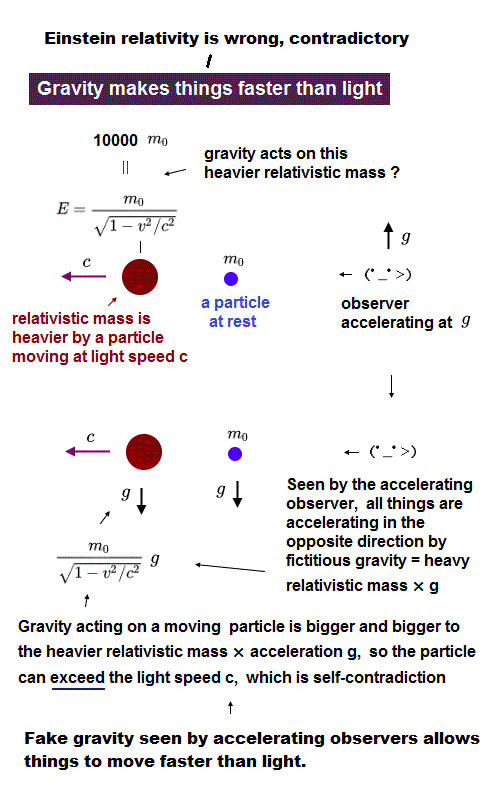Though several years have passed since Higgs discovery, our daily livings have NOT changed at all.
Higgs becomes a very convenient target for media and bloggers, and survives as "virtual" particle only inside news and blogs.

For example, after Nobel committee gave prize to Higgs boson, various quixotic news ( ex. God particle is destroying universe ? ) appeared, and confuse ordinary people.

Accelerators such as LHC are very expensive and built using big money and heavy tax from ordnary people.
But ordinary people had NO benefit from this "virtual" particle, generated ( ← ? ) faintly ONLY inside accelerator.

Even if two or three Higgs (?) bosons are found, the situation will NOT change at all.   NO benefit.
Unless Higgs can be utilized in our daily life, it's used Only as a "topic" for bloggers ( ex. Higgs contest ? ) and media.

## Higgs is generated Only one time in trillions of collisions ? ← Really found ?

(Fig.5)   Higgs boson is "too rare" (= 1/1000000000000 ) and unstable to be said "real".This site and this site say more than 1 trillion collisions are necessary to generate one Higgs boson. It's like looking for a needle in a haystack.

Futhermore, as shown on this site, Higgs lifetime is only 10-22 seconds ! Very unstable.
The standard model claims that Higgs is distributed all over the space around us, and gives mass to every object.

But if very rare and unstable Higgs produced in the accelerator is real, it cannot give mass to everything "stably".
If "infinite" Higgs exist around us, they must easily detect Higgs decay in our daily living ( without wasteful accelerator ).

## Higgs can be produced and detected without accelerator ?

(Fig.6)   Why do they need expensive accelerator ? ← meaningless.If the very unstable Higgs bosons in the accelerator are distributed all over the space around us, these Higgs are also unstable and must instantly decay into other particles.

If so, we can often detect boson (or fermion ) decay processes of Higgs in our daily life, NOT relying on accelerator.
But "infinite" Higgs around us cannot be found in our daily life, rare "Higgs" detected at LHC contradits original "infinite" Higgs which gives mass to everything.

Beta decay is NOT a rare phenomenon, in which heavy W boson (= 80 × protons ! ) is produced, they insist.
When this W boson is generated, Higgs must exist there, and give mass to W boson.

So in the process of ordinary beta decay, we can easily detect decay of W boson and Higgs in common laboratory. But neither of them can be detected.
It means W boson and Higgs, which appear ONLY inside accelerator, are different from the beta decay and original Higgs.

## Quasiparticle remains unreal and "fictitious", forever.

(Fig.7) Condensed matter physics relies on "fictitious" quasiparticles. ← "unrealistic", forever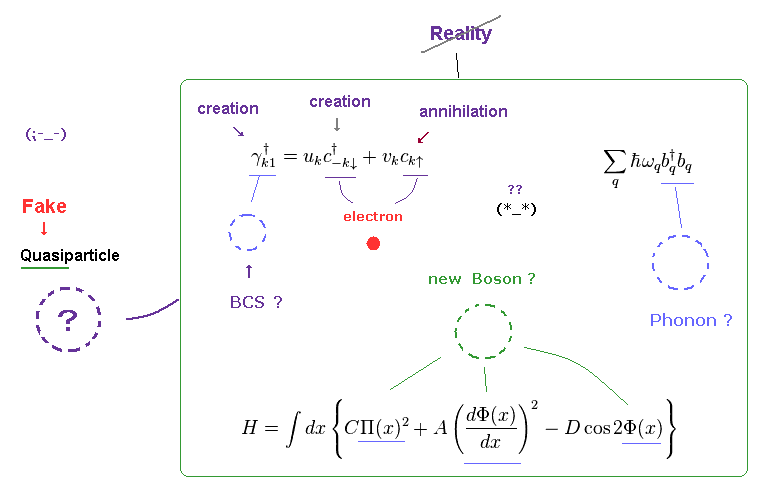Unfortunately, the current condensed matter physics was formulated in old days ( 1920 ~ 1960 ), which did NOT assume the present computer.

In spite of this tough situation, physicists at that time had to continue their research for better posts.
As a result, all they could do was create fictitious quasiparticle.

For example, phonon is another name for lattice vibration, so it's NOT a real particle.
This is the reason why they are called "quasi-particle".

Bogoliubov quasiparticle (= γ ) in BCS theory consists of creation and annihilation operators of electrons.
So these quasiparticles contradict the original definition of particle.

## Nobel prize should NOT be given to quasi (= fake ) particles !

### [ If Nobel prize is given to "fictitious" quasiparticle, the "elucidation" of truth will be prevented through many years. ]

(Fig.8) Even 2000 years from now, quasiparticles remain "fictitious" math operators.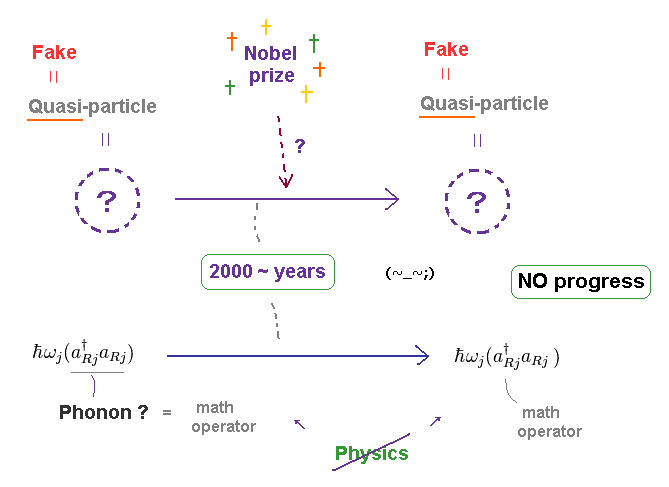Of course, in true "science", we have to continue to elucidate "truth".
So fictitious quasiparticle must be explained using real particles, as soon as possible.

Surprisingly, the current quantum mechanics tries to "intentionally" increase virtual quasiparticles ( see list ), going against the spirit of science.
Furthermore, they NEVER try to ask what these fictitious quasiparticles really are, forever !

So as long as we stick to this unreasonable quantum mechanics, even 2000 years from now, quasiparticle remains "fictitious" particle.
And very abstract math operators (= creation and annihilation ) have NO ability to describe concrete, dynamic motions of each particle, forever.

## Massless fermion, monopole, fractional-charge anyon are all "fictitious" quasiparticles.

(Fig.9) Massive electrons become massless !?   Anyon (= fractional charges ) is NOT a real particle.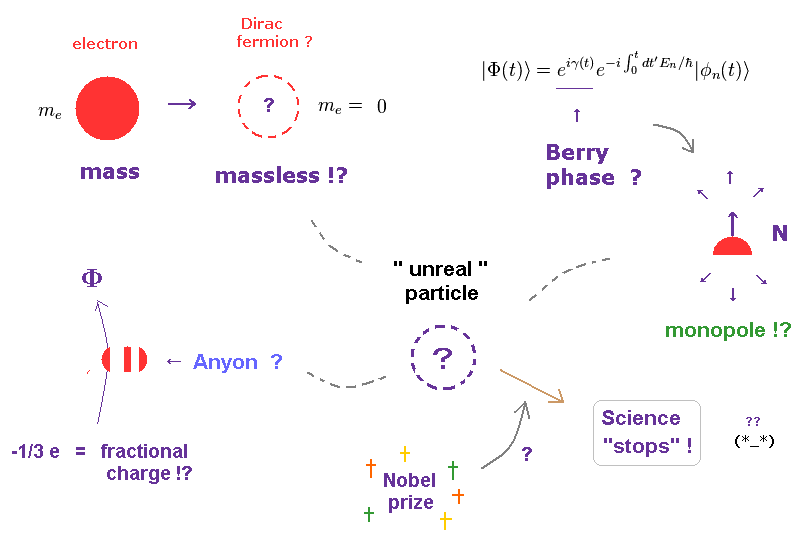As shown on this page, the current physics tries to create massless Dirac fermions using massive electrons.
They just call it "massless" Only from one experimental device (= ARPES ). Of course, these are NOT real particles.

They try to create "virtual" Berry phase and monopole to explain integer quantum Hall effect.
Furthermore, as shown this site and this site, they need strange anyons (= fractional charges !? ) to explain fractional Hall effect.

If Nobel committee gives prize to these fictitious quasiparticles, they continue to avoid asking what these quasiparticles really are, which means science will stop.

## Fantasy quantum mechanics "prevents" us from curing fatal diseases.

### [ As long as we believe in "unrealistic" quantum mechanics, your fatal diseases are "incurable". ]

(Fig.10)   Quantum mechanics obstructs the elucidation of "true" (= real ) microworlds.Quantum mechanics introduced in 1920s has been exposed to criticism due to its unreality such as superluminal spin and many-worlds (= Schrodinger's cat ).
Basically, quantum mechanics avoids clarifying clear mechanism of microphenomena and sticks to vague scientific attitude.

Due to its inability to compute multi-particle dynamics, quantum mechanics created fictitious quasi-particles and "imaginary" target of quantum computer, which state easily breaks within nanoseconds, completely impractical.

As you see, living things including our human bodies are highly sophisticated nano-machines, which is far beyond our current knowledge.
So, as long as we keep avoiding a clear picture of micro-phenomena, it is obviously impossible to cure various serious diseases such as cancer, HIV, ALS, severe allergy, dementia ..., forever.

## Quantum computer (= entanglement ) is "camouflage" to conceal uselessness of quantum mechanics.

(Fig.11) What is the true mechanism of superluminal entanglement ? → Shut up ! ( forever. )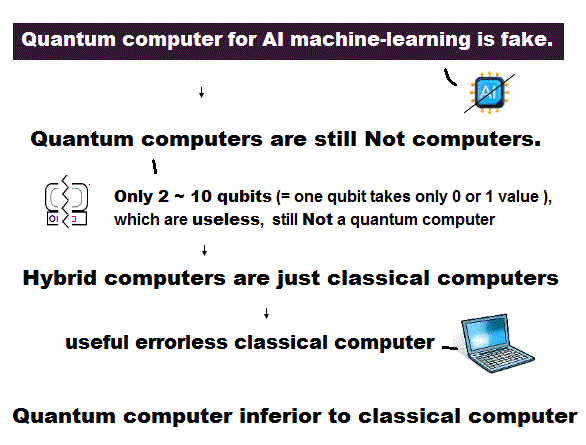As shown on this page, the coherent (= working ) time of superconducting qubits (= quantum comptuer !? ) is Only nano ~ microseconds even after very long-time researches.

So it is completely impossible to realize practical quantum computer, as long as they repeat the same patterns.
Trick of faster-than-light entanglement is fictitious "photon" particle.

The current researchers try to use this convenient word, "entanglement", whenever possible.
But superluminal link had NOT been proved inside very small qubits and solids ( ~μm ).

Judging from the fact quantum computer remains impractical despite extremely long researches, these "convenient" entanglement and quantum computer were created to conceal uselessness of quantum mechanics itself.

If Nobel prize is given to this ( superluminal ? ) "entanglement", the elucidation of true mechanism will be hampered.

## What are the shape and size of a "photon" ? → Shut up and calculate !

### [ Nobel committee should NOT admit ( virtual ) photons ! ]

(Fig.12) Photon is emitted from electron → its acceleration becomes "infinite" due to the sudden change.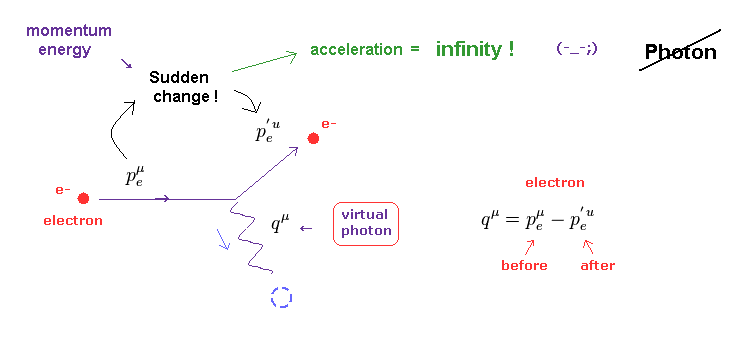As shown on this page, a photon is just an abstract math operator with NO physical entity ( ex. size and shape ).
So, if they give Nobel prize to photon-related things ( ex. quantum information ), the true nature of electromagnetic fields remains unknown.

Basically, according to the quantum field theory, the behavior of photon must be described using Feynman diagram.
Surprisingly, if the emitted ( or absorbed ) photon and electron satisfy the basic law of energy and momentum conservation, this photon always becomes virtual photon.

As shown on this page and this site, this virtual photon violates special relativity like "tachyon". This is the reason why it is called "virtual".

Furthermore, when a photon is emitted from ( or absorbed into ) an electron, this electron's momentum and energy suddenly changes.
This means the acceleration of electron at this time becomes infinity !   Impossible.

We have to consider more realistic view about lights emitted from electrons, using the whole field change and de Broglie waves, to avoid this contradiction.

## Photon spin is unrealistic, just classical polarization.

### [ They try to consider "everything" in relation to unreal "spin".   Why ? ]

(Fig.13)   Photon spin = circularly polarized light ?   Then, linearly polarized light ?As shown on this site, when electromagnetic wave is circularly porlarized, they just call its direction "spin".
So photon's spin means just the direction of classical light's polarization ( NOT fantasy spin ).

Then, common linearly polarized lights have NO spin !?
As shown on this site, an equal mixture of these right- and left-circular states yields a linearly polarized beam.

As you see, photon spin is too good and convenient to be true.
Actually, in this paper, the word of "polarized lights" can be often seen.

So, if Nobel prize is given to these fields related to photon (spin), the road to elucidation of truth will remain closed, and they continue trying to connect everything with unreal spin.

## If Nobel prize is given to unreal "spin", all scientific progress will be hampered.

(Fig.14)   Who needs unrealistic spin 1/2 and photon spin 1 ?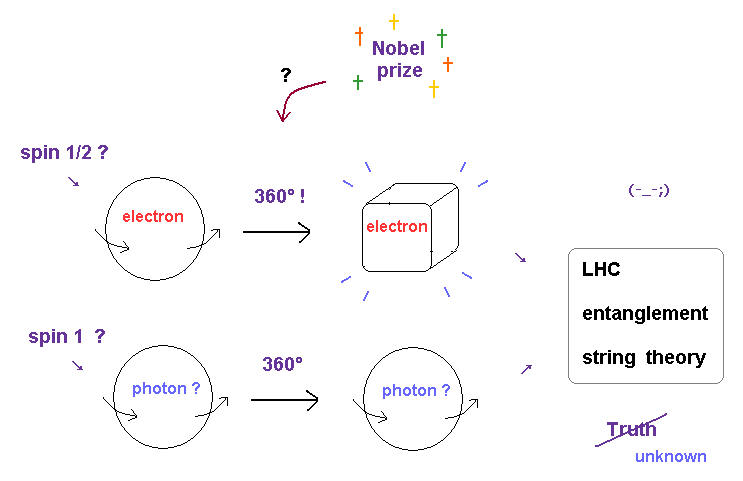As I said on this page, electron's spin 1/2 cannot return by 360 degree rotation, very unrealistic.
On the other hand, photon's spin 1 can return by one rotation ? So real ?

No, photon spin is just classical light polarization.   Then why they want to call it spin ?
Because the current quantum physics is restrained to this fictitious "spin", which prevents us from pursuing truth.

For example, if photon, virtual photon (= contradicts relativity ), spin don't exist, the foundation of standard model will be shaky.
Considering Higgs and SUSY are just used ONLY as "news items" ( ex. Scientific american ) for media, we do just fine without "spin".

Furthermore, as shown on this page, there are NO direct experimental results which showed photon "particle".
This imaginary photon is indispensable for particle physics, entanglement (= quantum computer, information ), and 10 dimensional string theory.

## Spin-Spin dipole interaction is too weak to explain actual data.

(Fig.15)   Spin-spin interaction energy is only 10-6 eV, which is easily broken at 0.05 K !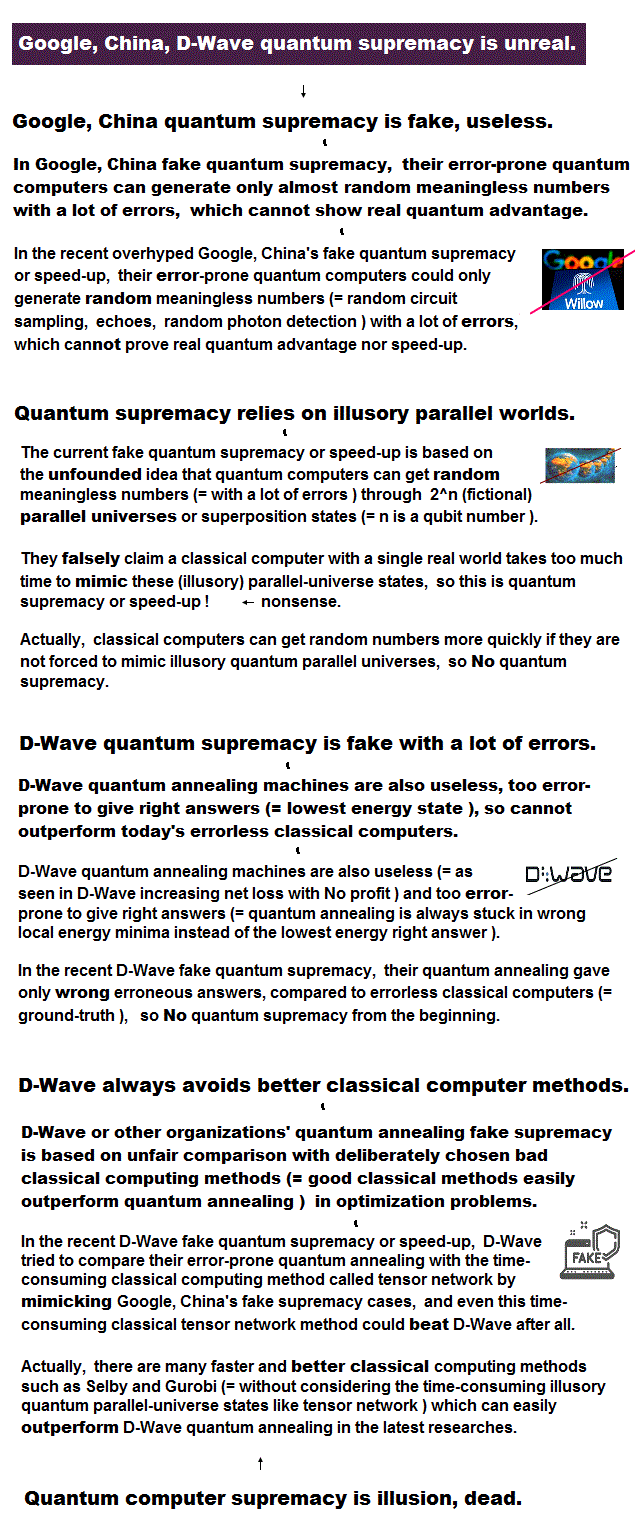As shown on this page and this site (p.7), spin-spin dipole (= Bohr magneton ) interaction enegy is too weak ( < 0.3 K in temperature expression ) to explain actual ferromagnetism ( 1043 K in Fe temperature ! ).

For example, the bond length between Fe-Fe is about 2.8 Å (= 2.8 × 10-10 meter ). See this site (p.2).
Using this page, this site, this site (p.6), we find spin-spin dipole interaction becomes only 4.55 × 10-6 eV (= 0.05 K ! ).

Pauli exclusion principle cannot be explained by too weak spin-spin interaction, either.
As shown on this site, lithium with 3 × 1s configuration gives lower (= 8.46 = -230 eV ) than experimental value (= -203 eV )

This wide discrepancy ( 27 eV = 230 - 203 eV ) shows this Pauli repulsive force is caused by other strong effects such as de Broglie wave interference.

Furthermore, sodium D line splitting is too wide to be explained by spin-orbit coupling.
Nobel prize should NOT be given to the wrong concepts (= spin ) contradicting experimental results.

## Many-worlds, Copenhagen interpretations ... "unreal" wavefunctions.

(Fig.16) Schrodinger's wavefunction = many-worlds or "superluminal" collapse !?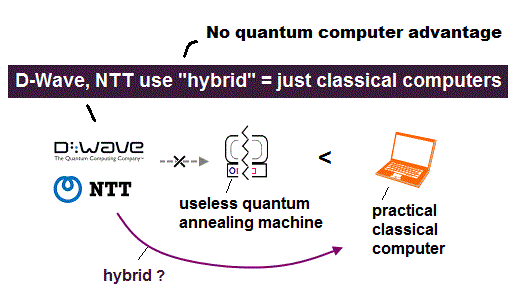Schrodinger equation in quantum mechanics only presents "vague" probability wavefunctions.
So, the moment we try to observe its electron, a "superposition" consisting of various states reduces to a single electron (= collapse ).

The speed of this wavefunction collapse is "infinite" (= faster-than-light ), which idea is the origin of spooky link, entanglement.
In standard Copenhagen interpretation, the observer must have very "special" power to cause this wavefunction collapse. It is very unnatural.

So unrealistic Many-worlds interpretation was introduced, and dominates in the field of quantum mechanics.
In many-worlds, when the observer tries to observe the electron, he just sees one of many states (= worlds ), in which collapse speed does not exceed "c".

Of course, this many-world theory is very "ad-hoc" and impossible from the realistic viewpoint.
But quantum mechanics cannot treat "real" electron and wave (= Pilot wave ).

## Real electron and wave are "impossible" in quantum mechanics.

### [ de Broglie-Bohm (= pilot wave ) theory is impossible. ]

(Fig.17) Angular momentum = 0 → electron "collides" with nucleus ? Wave is cancelled out ?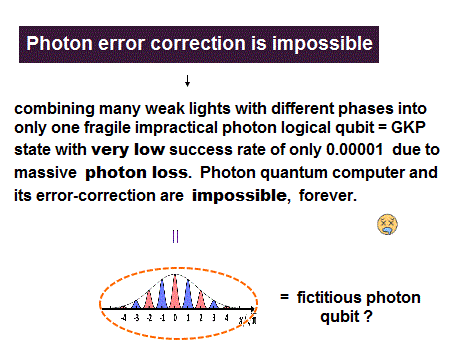As shown in Schrodinger wavefunctions of hydrogen, quantum mechanics always needs unrealistic state of angular momentum L = 0, in which state, the electron always crashes into nucleus ?

All wavefunctions are based on de Broglie wave relation.
But if this angular momentum zero is real, these de Broglie waves are cancelled out by themselves due to destructive interference in linear motion.

So, the idea of "real" electron and wave are impossible in quantum mechanics.
This is the reason why pilot-wave (= de Broglie-Bohm ) theory was obsolete, and has NOT been accepted.

## Reason why Schrodinger's hydrogen is wrong.

### [ "Negative" kinetic energy ( Tr < 0 ) is unrealistic. ]

(Fig.18) Schrodinger's 2P "radial" wave function ( angular momentum = 1 ).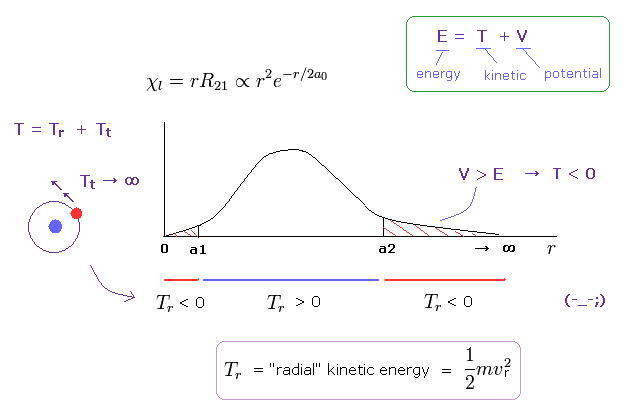For example, in Schrodinger's 2P states, the radial kinetic energies ( Tr = 1/2mv2 ) become negative in both sides as shown in Fig.18.
In the region of a2 < r, potential energy V becomes higher than total energy E.
As a result, kinetic energy must be negative ( ← Impossible ! ).

In the region of r < a1, tangential kinetic energy ( =Tt ) becomes unrealistically larger.
To cancel this increase, radial kinetic energy (= Tr ) must be negative. ( ← NOT tunnel effect, because V < E. )

Again, this is the reason why real wave theory (= pilot ) is impossible, and physicists escape into fantasy many-world interpretation.

## Bohr's accelerating electron does NOT radiate energy.

(Fig.19) Textbooks about Bohr model are completely wrong.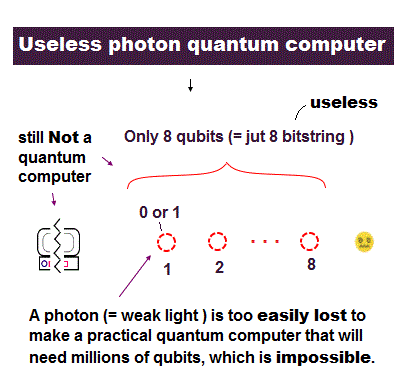Unfortunately, Bohr model electron does NOT fall into nucleus, as shown on this page.
Most people are deceived by wrong explanations in standard textbooks and websites.

It uses Poynting vector (= E × H ) as the energy flow. ( See this and this )
This Poynting vector is equal to the change of the electric and magnetic energies in the vacuum.

This electric energy in the vacuum (= 1/2εE2 ) means the potential energies needed to store infinitesimal minus (or plus) charges to the spherical conductor (= Fig.20 left, or see this, this, this ).

## A single electron is NOT a spherical conductor made of "infinitesimal" charges.

(Fig.20) Bohr model electron is falling into nucleus ? ← Mind-control by textbooks !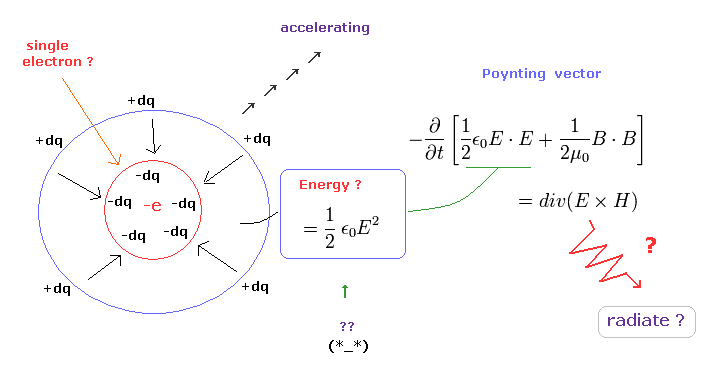But a single electron is NOT made from smaller charges. ( A single electron is the smallest charge. )
There is NO concept such as "electric energy" around a single electron.

It means the vacuum electric energy (= 1/2εE2 ) in a single electron is NOT energy, as a result, Poynting vector itself is meaningless in this single electron's case.

So this wrong explanation is a kind of brainwashing about Bohr model by quantum mechanical textbooks.
Only when more than one charges are involved, they can radiate energy.

## Three body problem (= helium ) is very complicated.

(Fig.21) Helium atom is NOT a simple wavefunction combining two hydrogens.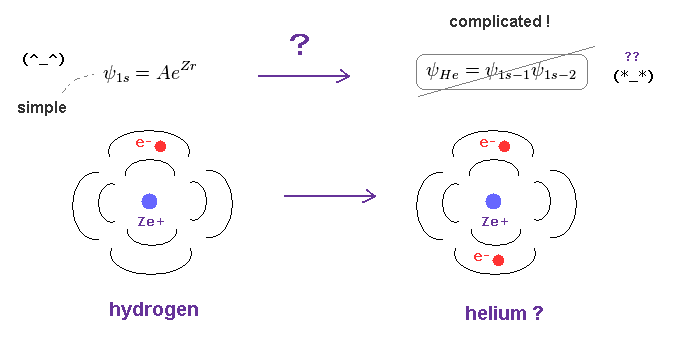In almost all textbooks of quantum mechanics, Only the wavefunction of hydrogen atom is seen.
But unless we can handle multi-electron atoms, we cannot apply quantum mechanics to actual science.

In fact, even a simple helium atom cannot be solved analytically.
Helium is three-body problem, which wavefunction (← NOT determined ! ) is unrealistically complicated.

Two electrons of helium atom are always repelling and avoiding each other.
Due to this complicated motions, helium wavefunction cannot be constructed just by fusing two hydrogen functions.

## Even simple helium atom has NO "exact" solution.

(Fig.22) Repulsive realtion between two electrons makes wavefunction very hard to treat.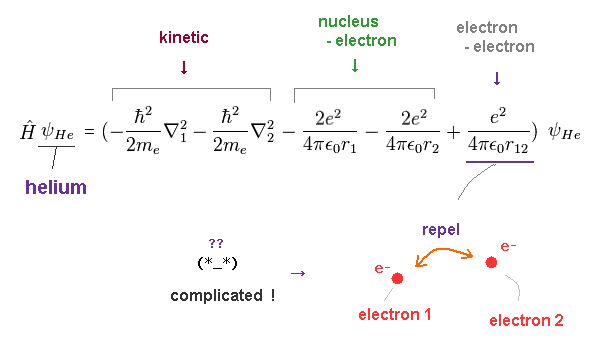Schoridinger equation of helium atom consists of three parts:   kinetic energy, potential energies between each electron and nucleus, and repulsive potential energies between two electrons.

(Fig.23) Distance between two electrons.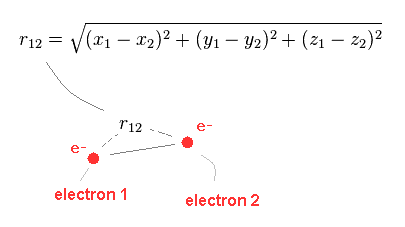Here r12 denotes the distance between two electrons.
The existence of this variable makes helium equation very hard to treat.

## If helium consists of two hydrogen wavefunction .. ?

(Fig.24) Helium atom = hydrogen 1s + hydrogen 1s wavefunctions ?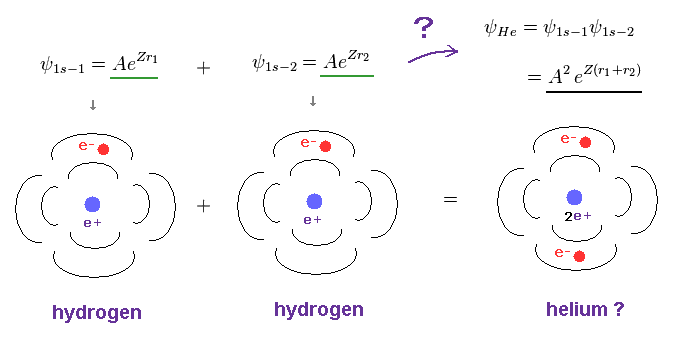In quantum mechanics, both of two electrons of helium are also in 1s states ( with NO angular momentum ! ).
First, we consider helium's wavefunction consists of two hydrogen 1s wavefunction ( 2 × ψ = A eZr ).

Here the exponential parameter (= "Z" ) is a variational charge parameter ( in hydrogen, Z = 1 ).
Substituting the wavefunction of Fig.24 into Fig.22, we have

(Fig.25) The calculation value (= -77.49 eV ) is higher than true ground state energy.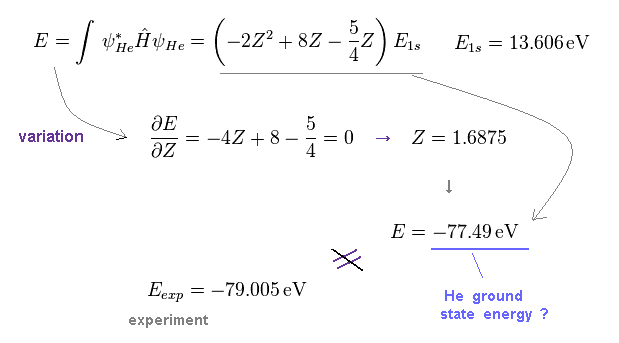As shown on this site and this page, when we vary the parameter Z to minimize the total energy E, the calculated value becomes -77.49 eV.

This value is higher than the true ground state energy (= -79.005 eV ).
Because the wavefunction of Fig.24 does NOT consider the "repulsive" motions of two electrons.

(Fig.26) Effective charge of helium atom = 1.6875 ?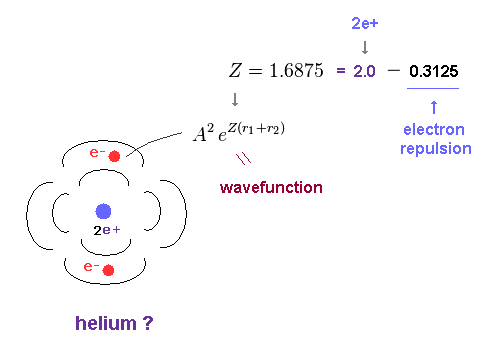As shown in Fig.25, when the effective charge Z is 1.6875, total energy E becomes the lowest.
The difference (= 0.3125 ) between 2.0 (= helium nuclear charge ) and 1.6875 is caused by the repulsive effect of another electron.

## We have to consider "repulsive" effects of two electrons.

(Fig.27) Two electrons are "actually" avoiding each other.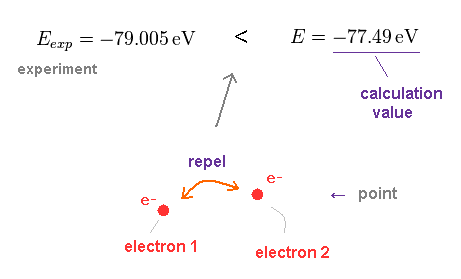As shown in this result, you can find two electrons of helium are "actually" repelling and avoiding each other, which causes this difference in energies.

## Wavefunctions are very "bad" at handling "avoiding" electrons.

(Fig.28) Two "spreading" probability wavefunctions can describe repelling electrons ?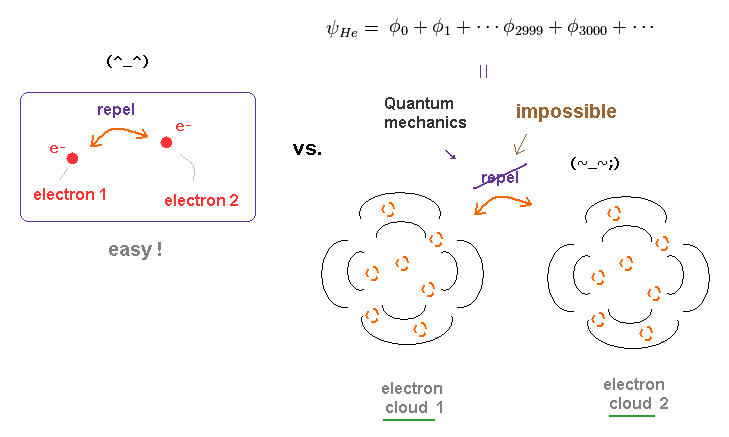According to the quantum mechanics, Schrodinger wavefunctions mean "probability" density, and must be spreading all over the space.

Different from usual electromagnetism, it is much more difficult to describe avoiding electrons using these spreading wavefunctions.
Thinking commonsensically, it is impossible for two wavefunctions spreading all over the space to avoid each other.

So even in a simple helium atom, quantum mechanics needs more than 3000 "complicated" terms (← impractical ! ) , as shown on this site.

## Variational method of quantum mechanics is very "artificial".

(Fig.29) It's "impossible" to try all kinds of variational functions and know exact values.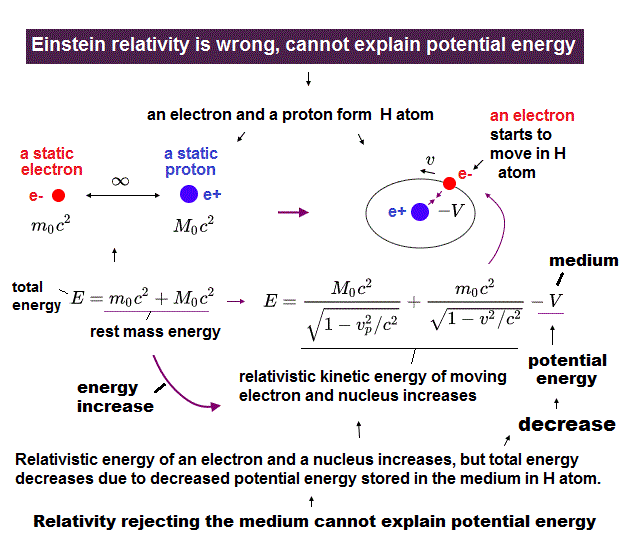As shown in Fig.24, Schrodinger's wavefunctions do NOT consider repulsive effects between electrons.
So the calculated energies are always higher than true ground state energy.

So physicists try to lower this energy using variational methods and complicated functions such as Hylleraas and configulation interaction (= CI ).
As I said, these wavefunctions need more than 3000 terms even in simple helium atom. Very impractical.

Though they argue this variational method can give exact ground state energy, these results are very doubtful.
Because it is impossible to try all kinds of trial wavefunctions.

So we cannot know the exact lowest values based on this variational methods.
In fact, in this paper (p.3), they fix the exponential parameter at k = 2.0 to get exact values.

If they use multi-exponential parameters and vary them independently, they would be able to get lower energy.
In this paper, they optimize exponenteial parameter in quasirandom (= NOT variational ) manner to get the lowest energy.   This is strange.

## Energy conservation law is "violated" in quantum mechanical atoms !

### [ Stationary wave functions → repulsive energy between two electrons increases to infinity ! ]

(Fig.29')   Helium wave functions "disobey" energy conservation at any points.As helium atom contains two electrons, the equations of helium cannot be solved.
So we have to choose some approximate trial wavefunctions for helium.

The important point is that energy conservation law is violated in all atoms and molecules in quantum mechanics except for hydrogen, if their wave functions represent "stationary" probability.

In stationary wavefunctions, the kinetic energies and potential energies between nucleus and electron at each point are constant.   But the repulsive energy between two electrons changes depending on the distance (= r12 ) between two electrons.

Surprisingly, when the distance between two eletrons are close to zero, the potential energy increases to infinity !
So depending on positional relationship between two electrons, total energy of helium changes, which shows total energy conservation is violated.

In solvable hydrogen atom, of course, total energy (= H ) is always constant (= conserved ) in any position of an electron.   So quantum mechanics cannot deal with many-body atoms. Only this model satisfies energy conservation in any positions in the specific orbits.

## Quantum equations cannot handle many-body system → produced many "wrong" concepts.

(Fig.30)   Complicated Schrodinger equation + determinants cannot handle large molecular system.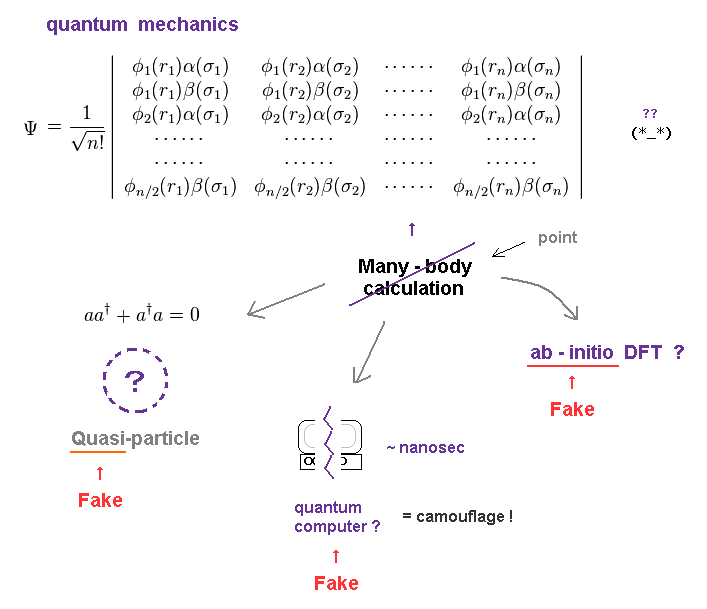For example, for two electrons to avoid each other even in simple helium atom, quantum mechanics needs more than 3000 terms ( ← impractical ! ), as shown on this site.

So, the current quantum mechanics cannot handle larger molecules, different from the insistence of various sites.

See very interesting paper, as follows,
----------------------------------------
p.5. suggesting that first principles is favored by the density functional theory ( DFT ) community since in this kind of work it is quite clear that some semi-empirical elements are introduced.

p.6. Regarding current ab initio calculations it is probably fair to say that they are NOT really ab initio in every respect since they incorporate many empirical parameters.
------------------------------------------

It is obvious that all these fictitious quasiparticle, ab-initio (← ? ) DFT, and quantum computer were produced to conceal uselessness of quantum mechanics.

## "Ab-initio" density functional theory ( DFT ) is completely a fake !

(Eq.1) Density functional theory is NOT ab-initio.Though the present physical society likes to use the name of ab-initio density functional theory (= DFT ), this given name is clearly fake.
As I said above, the most complicated parts are correlation (= repulsive electrons ) functions.

As shown on various sites ( this, this (p.10), this (p.1) ), these exchange-correlation functionals are unknown, and have to be determined artificially.

So, the name of "ab-initio DFT" or first-principle is a fake, which was introduced to conceal the inconvenient facts.
Actually this site (p.23) says DFT is just a semi-empirical (= NOT first-principle ) method.

## Even the simplest local density approximation ( LDA ) is NOT ab-initio.

(Eq.2) LDA - VWN correlation functional contains "artificial" paremeters.As shown on this page, even the simplest local density functional (= LDA ) uses artificially adjusted correlation functionals, which means NOT ab-initio.

On this site p.4, there are several expressions for LDA correlation energy which have been obtained by fitting to the results of accurate QMC calculations.
Most popular choice in LDA correlation functions is VWN-LDA as shown in Eq.2.

ε of Eq.2 is on this site (p.9).
This LDA correlation function contains as many as 12 fitting parameters.
So it is NOT first-principle at all.

## How "spreading" wavefunctions can describe two repulsive electrons ?

### [ Wavefunctions with different charges Z can avoid each other !? ]

(Fig.31) Superposition of two H wavefunctions with different charge Z ?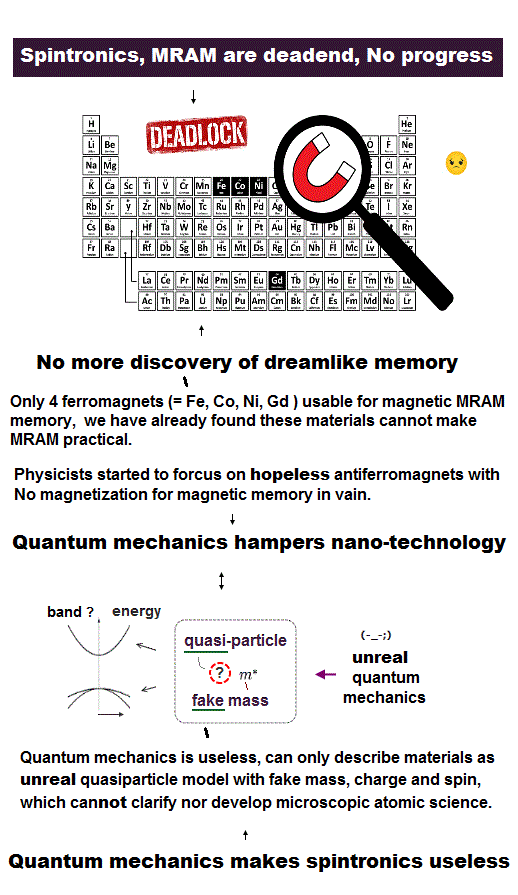As I said, it is extremely hard to describe two electrons avoiding each other using "spreading" wavfunctions.
One of methods is configuration interaction (= CI ).

As shown in Fig.31, if a helium atom consists of two hydrogen wavefunctions with different charge Z ( ex. Z1 =1.453, and Z2 = 2.91 ), these two wavefunctions can slightly avoid each other.

## Effective nuclear charge is greater than Z = 2 ?   Impossible !

(Fig.32) "Superposition" can describe two electrons avoiding each other ?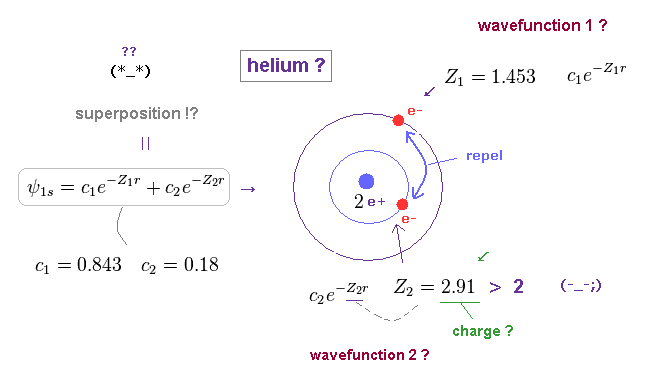Using this site, you can try various charges Z to get He energy.
When two charges Z are 1.45 and 2.90, the calculated value becomes the lowest ground state energy (= -77.87 eV ).

Of course, this value does NOT reach the exact energy (= -79.005 eV ) at all, so they have to add different kinds of wavefunctions ( ~ 3000 terms ! ).

As you notice, the charge of Z = 2.91 is unrealistic, because it exceeds helium nuclear Z = 2 !
So the helium wavefunction is an "unreal" concept with NO physical meaning.

-------------------------------------------
It should be noted that the energy minimum occurs at a value of k which is greater than Z.
Thus, the interpretation of this parameter as a "effective nuclear charge" is NO longer valid.
-----------------------------------------

(Fig.33) Repulsive Coulomb energy between two electrons becomes lower ?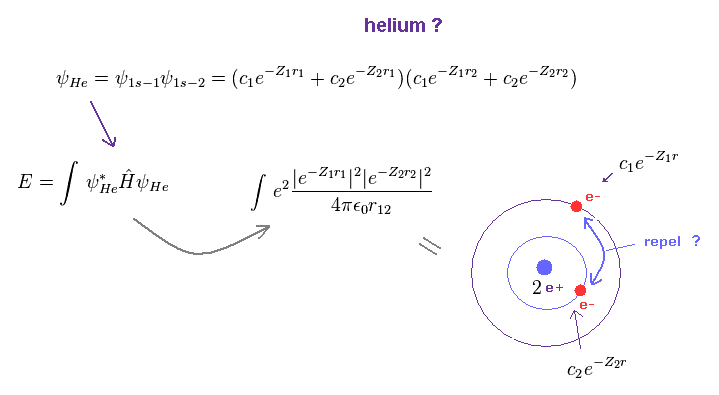As you see in Fig.33, this superposition wavefunction can make repulsive Coulomb energy lower than the original one.
Because these two Z parameters are different, overlapping parts of two wavefunctions are reduced.

## But this superposition wavefunction is unreasonable.

(Fig.34) When the wavefunction contracts (= Z is bigger ), how potential energy changes ?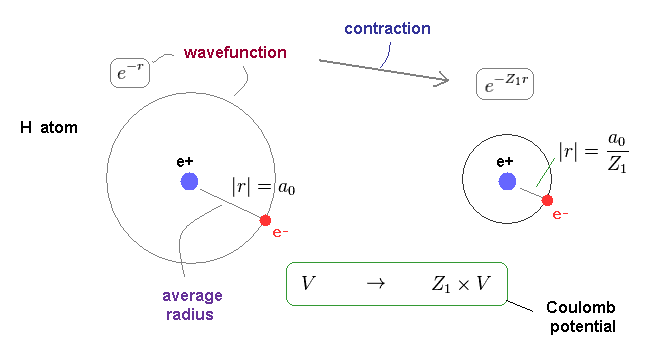But this "superpositon" wavefunction is very unreasonable and impossible from realistic viewpoint.
As shown in Fig.34, when the effective Z becomes larger ( 1 → Z1 ), this wavefunction becomes contracted.

The average radius becomes shorter by × 1/Z1.
So the potential energy (= V ) also becomes lower by this ratio.

## Kinetic energy becomes too large by this contraction.

### [ Kinetic energy increases in proportion to a "square" of Z. ]

(Fig.35) Kinetic energy increases too much !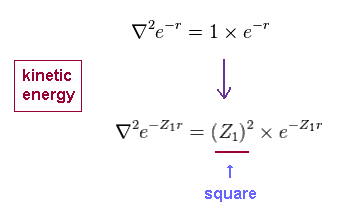All information about electron's kinetic energy is included in the wavefunction.
As you see Fig.35, this kinetic energy increases too much in proportion to a square of Z1.

(Fig.36) Wavefunction contracts → Kinetic energy (= T ) increases in proportional to Z2.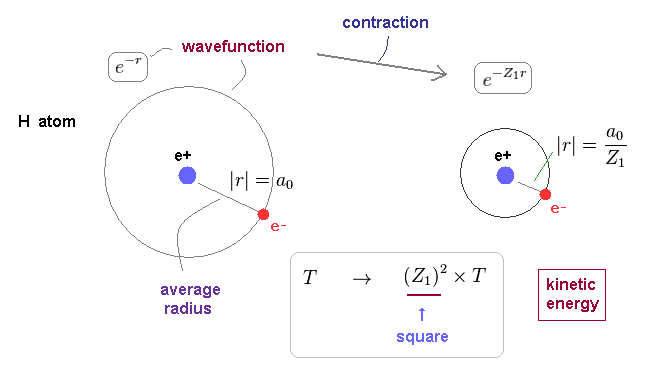As shown on this page, this is the mechanism of variational method in which equilibrium state ( satisfying Virial theorem ) becomes the lowest in energy.

(Fig.37) Toal energy becomes much higher ( 1/2 V → zero !? ).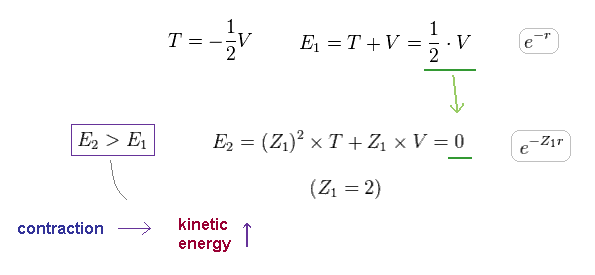Here we suppose when Z = 1, this wavefunction satisfies Virial theorem ( T = -1/2V ).
From Fig.34 and Fig.36, the total energy E2 becomes much higher than the original E1.

For example, when the wavefunction contracts obeying 1 → Z1 = 2, total energy becomes zero (← NOT negative, impossible ! )

So the idea of varying exponential parameter Z contradicts the realistic electron's motions.
Because these superposition wavefunctions with different Z do NOT satisfy energy conservation law.

## He wavefunction changes depending on positional relationship ?

### [ Hylleraas function contains interelectronic distance. ]

(Fig.38) r12 = distance between two electrons.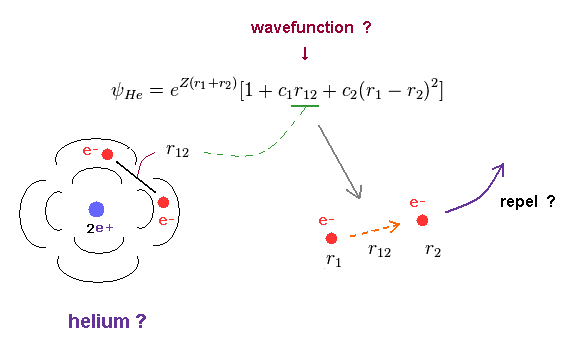There is another way to express two avoiding electrons.
Hylleraas trial function contains the distance (= r12 ) between two electrons.

This method is very hard to compute, so it can be applied only to small atoms such as helium and lithium.
If you see Lithium case, you easily find this method is completely impractical and cannot be used in larger atoms.

## Wavefunction = probability is false in this function.

### [ "Stationary" probability wavefunctions are illusions ! ]

(Fig.39) Even when electron 1 stays at the same positon, probability changes in helium ?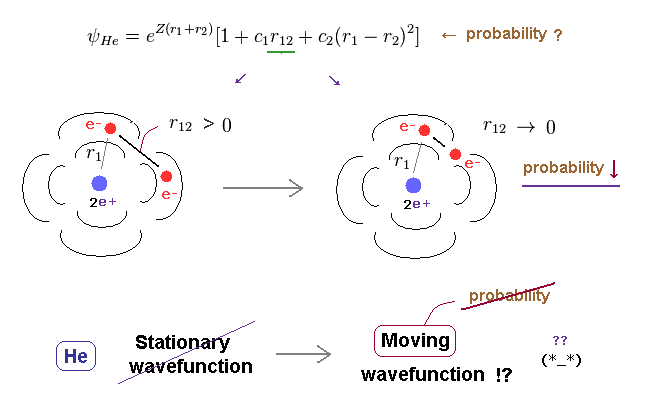Even when the electron 1's position remains the same, the whole probability changes depending on another electron's position.
Because this wavefunction includes interelectronic distance (= r12 ).

This means if we try to express repulsive electrons, we have to give up the idea such as "stationary" wavefunctions or probability.

## More than "3000" terms are needed to get exact values. ← Very impractical.

(Fig.40) Hylleraas function + variational method are unrealistically complicated.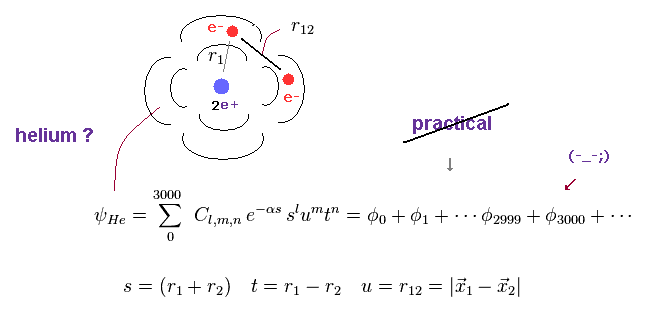As I said, more than 3000 terms are necessary even in a simple helium atom.
This functions contains "s", "u", "t" variables.

So as the distance between two electrons are shorter, the whole probability becomes smaller, which can describe two avoiding electrons, they insist.

But unfortunately, this Hylleraas functions do NOT handle "true" electron's motion, either.
For example, even when r12 gets shorter, if two electrons are at just the opposite sides of nucleus, its energy becomes lower.

So from the realistic viewpoint, we have to consider the relationship among two electrons and nucleus ( NOT only electrons ). Of course, complicated wavefunctions cannot handle this situation.

## Quantum mechanics cannot compute nuclear movement (= reduced mass ) correctly.

(Fig.41) Calculation value is lower than experimental value. ← Reduced mass effect ?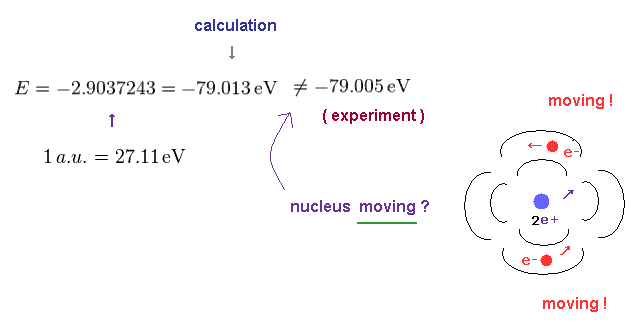As shown on this site, the computed value of He energy becomes -79.013 eV, which is lower than experimental value (= -79.005 eV ).

They say this difference is caused by nuclear movement ( reduced mass effect ).
But the problem is that quantum mechanical wavefunction cannot predict correct reduced mass.

## Reduced mass of helium is NOT simple.

(Fig.42) There are a lot of different reduced mass (= μ ) in helium atom.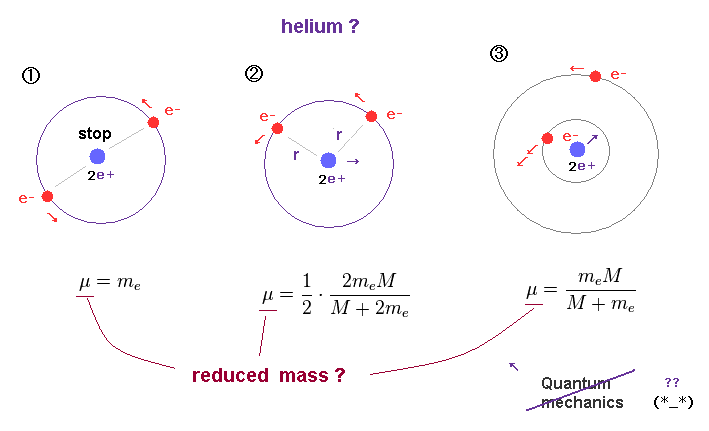For example, in Fig.42 left, two electrons are moving at the opposite sides of nucleus.
In this case, helium nucleus is stationary, and reduced mass remains the original electron mass.

In Fig.42 middle, distances between each electron and nucleus are the common "r".
In this case, we have to use "2m." (= m + m ) to get reduced mass.

In Fig.42 right, only one of electrons is very close to nucleus.
In this case, another electron, which is very far away from nucleus, has almost NO influence on nuclear motion.

So in the last case, reduces mass must be gotten using "m" instead of "2m".
Unfortunately, quantum mechanical helium cannot discriminate these different reduced mass, which means computed value is very doubtful.

## Basically, "spreading" wavefunctions cannot describe two electrons avoiding each other.

(Fig.43) If two probability wavefunctions try to avoid each other, it becomes unrealistically complicated.Basically, the wavefunction was originally introduced to express "vague" probability density.
So it is always spreading all over the space, even in one-electron hydrogen atom.

As you see in Fig.43, it is very unreasonable and unnatural for these spreading wavefunctions (= electrons clouds ) to try to avoid each other.

This is the reason why the Schrodinger wavefunctions of multi-electron atoms becomes unrealistically complicated.
Ordinary classical electrons are quite natural and much simpler to describe these repulsive Coulomb effects.

## Variational methods in helium are very doubtful.

(Fig.44) Exponential parameter "k" is fixed at 2.0 → Variational method !?Of course, it is impossible for us to try all kinds of trial functions.
So we cannot know the exact lowest values of variational methods.

In this paper (p.3), the exponential parameter k is fixed at 2.0, but they can obtain exact energy.
"Fixed" parameter means this is NOT a variational method.

Furthermore, if we vary this parameter "k" or increase the kinds of "k" like Fig.28, this computed value can be much lower than this result.   So this result is doubtful and NOT final.

In this case, the effective charge Z is "1", which is an unrealistic helium.
( Compare this with Z = 1.6875 in Fig.26 ).

(Fig.45)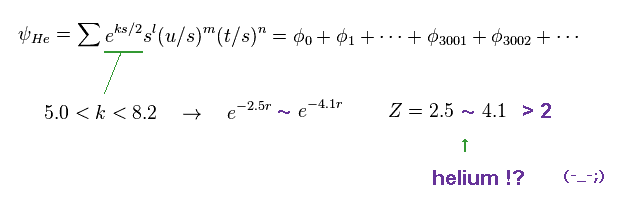In this paper, they limit this exponential parameters to 5.0 < k < 8.2 in case of different-type of trial functions.
What does this discrepancy between k = 2.0 and k > 5.0 mean ?   These choices are very artificial.

Also in this case, effective charge Z ( > 2.5 ) becomes larger than helium nucleus "2".
Again, unrealistic helium atom.

## Ab-initio quantum chemistry is NOT ab-initio.

(Fig.46) Slater-type wavefunctions.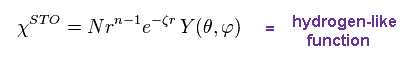Ab-initio Hatree-Fock, CI methods start from choosing basis sets (= wavefunctions ).
Slator-type wavefunction are equal to hydrogen atom, as shown on this site .

All energy levelds are the same both in Schrodinger and Bohr model.
This is the reason why the current quantum chemistry gives values close to experimental values.

## There are NO physical meaning in multi-electron wavefunctions.

(Fig.47) Exponential parameter = effective charge is real ?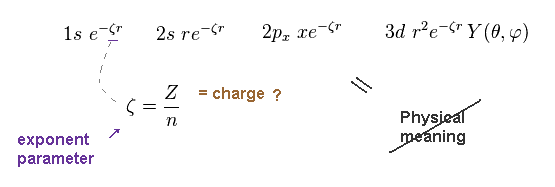Basically exponantial parameter (= ζ ) expresses effective charge Z.
But as I said in Fig.32 and Fig.45, this effective charge can exceed actual nuclear charge.

So multi-electron wavefunctions have NO physical (= realistic ) meanings at all.
These are just "mathematical" tools.

(Fig.48) Gaussian-type wavefunctions.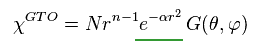Though Slater-type wavefucntions can give more exact energies, they cannot be used in larger atoms.
So gaussian-type functions ( =GTO ) were produced.

## Each parameter is fixed ← NOT ab-initio.

(Fig.49)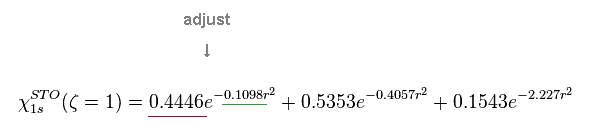They optimize all parameters (= coefficients and exponents ) to mimic Slater-type functions using Gaussian functions.
As shown on this site (p.6) and this site (p.18), all these paramters are fixed in calculating molecules.

This is one of reasons why "ab-initio" quantum chemistry is NOT true.

(Fig.50) Exponents of hydrogen is different from the original atom.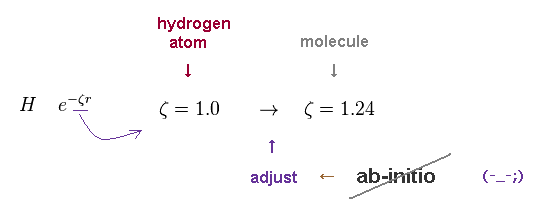The exponential parameter of the original hydrogen atom is "1".
But in most basis sets, they artificially change this parameter into 1.24 by fitting to molecular bonding.

Again, this preparation means it is NOT an ab-initio method.

(Fig.51) Fixed (= contracted ) basis set is NOT ab-initio.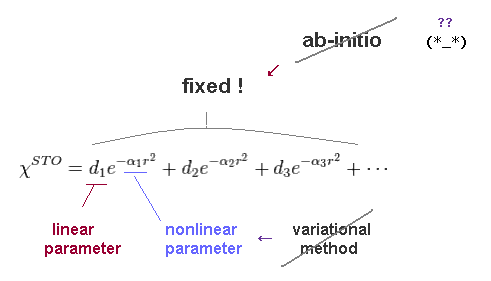As shown on this site (p.6) and this site, "ab-initio" quantum chemistry is NOT a "ture" ab-initio.
They just choose appropriate basis set for each atom and molecules.

Basically, variational calculation of exponential paramters (= α ) is hard and impossible, when several different variables are used ( see this site (p.19) ).
( Only coefficient, linear parameters can be calculated variationally. )

Of course, as more different kinds of exponential variables are used, we can lower the energy.
Due to the limitation of trial functions, it is impossible to confirm variational method is really correct or not.

(Fig.52) 6-31G, double zeta functions. NOT ab-initio.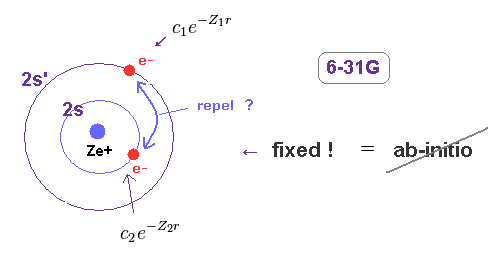For example, in 6-31G basis set, they use two different expoential parameters in the same 2s functions.
Because, as I said in Fig.31, as more different exponetial parameters are used, repulsive energy can be lower.

Using basis set prepared beforehand means this is NOT a true ab-initio.

## Polarization and diffuse functions are artificial.

(Fig.53)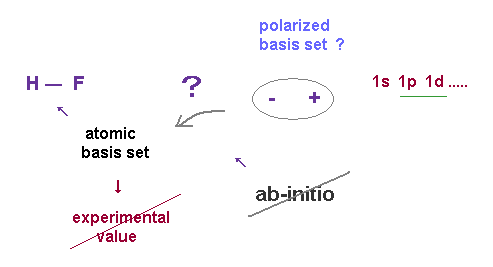Basically, if we use only the original atomic functions, we cannot come close to the exact energy.
So depending on the situation, we add polarization and diffuse functions to them. ( ex. unreal "1p" state )

Again, this is just artificial manipulation, and NOT ab-initio.
Furthermore, overestimation of energy (= basis set superposition error ) can happen, as shown on this site (p.4)

## Nobel committee should muster up the courage to make decisions.

### [ You should recall many great figures "disagreed" with the current physics. ]

(Fig.54) Nobel prize is a "golden" opportunity to correct basic errors.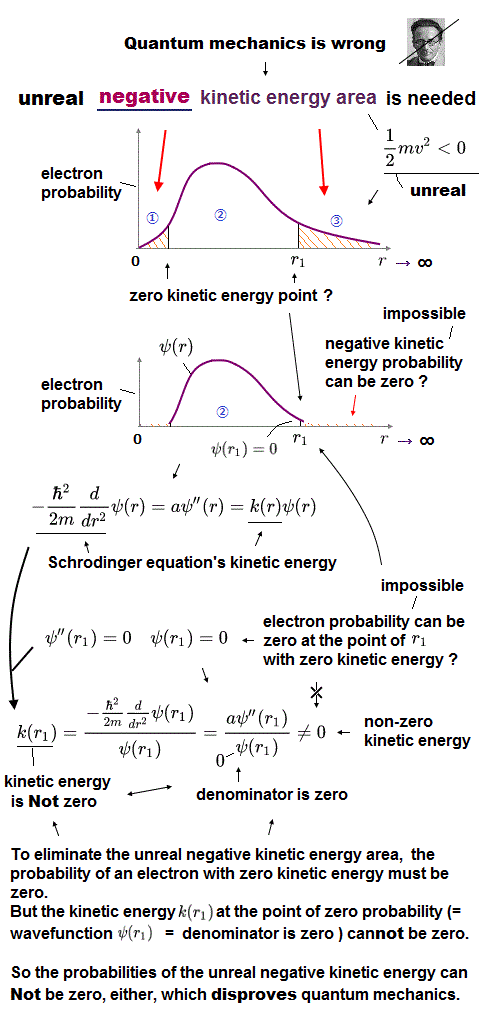As a consequence of the present quantum mechanics and relativity, Only 10-dimensional string theory is left as unified theory, though it is clearly unrealistic. It's disastrous for newcomers in physics.

In fact, the present quantum theory did NOT gain the approvals of great figures in the physics.
Einstein and Schrodinger resolutely opposed the unrealistic quantum mechanics.

And Dirac was very unsatisfied with the quantum electrodynamics (QED), which gives infinity.
Feynman disliked the 10-dimensional string theory.

But other quantum gravity theories such as loop quantum gravity (LQG) failed as an unified theory.
( Persons involved in LQG should NOT make new students "entertain" excessive hope, which would surely blast their careers. )

So only the string theory is left. ( Though, of course, 10 dimension is impossible in this real world. )
In this very paradoxical situation, there is ONLY one choice left.

We have to return to the early quantum physics and correct them.
( Because the string theory completely depends on the quantum mechanics and relativity. )
As shown on top page, all these miserable situations were caused by the very complicated three-body problems in 1920s.

Nobel committe should take courage to correct these basic errors indicated by the great figures in physics.
Only the most influential Nobel prize has the power to do it.2014/9/17 updated. Feel free to link to this site.